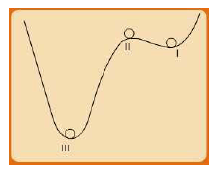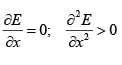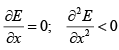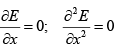Types of Thermodynamic Equilibrium

# Types of Thermodynamic Equilibrium - Thermodynamics - Mechanical Engineering

Types of Thermodynamic Equilibrium

Thermodynamic Equilibrium In general change of state of a thermodynamic system results from existence of gradients of various types within or across its boundary. Thus a gradient of pressure results in momentum or convective transport of mass. Temperature gradients result in heat transfer, while a gradient of concentration (more exactly, of chemical potential, as we shall see later) promotes diffusive mass transfer. Thus, as long as internal or cross-boundary gradients of any form as above exist with respect to a thermodynamic system it will undergo change of state in time. The result of all such changes is to annul the gradient that in the first place causes the changes. This process will continue till all types of gradients are nullified. In the ultimate limit one may then conceive of a state where all gradients (external or internal) are non-existent and the system exhibits no further changes. Under such a limiting condition, the system is said to be in a state of thermodynamic equilibrium. For a system to be thermodynamic equilibrium, it thus needs to also satisfy the criteria for mechanical, thermal and chemical equilibrium.

Types of Thermodynamic Equilibrium

A thermodynamic system may exist in various forms of equilibrium: stable, unstable and metastable. These diverse types of equilibrium states may be understood through analogy with a simple mechanical system as depicted in fig. 1.3 – a spherical body in a variety of gradients on a surface.Fig. 1.3 Types of Mechanical Equilibrium

Consider the body to be initially in state ‘I’. If disturbed by a mechanical force of a very small magnitude the body will return to its initial state. However, if the disturbance is of a large magnitude, the body is unlikely to return to its initial state. In this type of situation the body is said to be in unstable equilibrium. Consider next the state ‘II’; even a very small disturbance will move the body to either positions ‘I’ or ‘III’. This type of original equilibrium state is termed metastable. Lastly, if the body is initially in state ‘III’, it will tend to return to this state even under the influence of relatively larger disturbances. The body is then said to be in a stable equilibrium state. If ‘E’ is the potential energy of the body and ‘x’ is the effective displacement provided to the body in the vertical direction, the three equilibrium states may be described by the following equations:

Stable Equilibrium:....(1.7)

Unstable Equilibrium :....(1.8)

Metastable Equilibrium:....(1.9)

The above arguments may well be extended to understand equilibrium states of thermodynamic systems, which are relatively more complex in configuration. The disturbances in such cases could be mechanical, thermal or chemical in nature. As we shall see later (section 6.3), for thermodynamic systems, the equivalent of (mechanical) potential energy is Gibbs free energy. The considerations of change of Gibbs free energy are required to understand various complex behaviour that a thermodynamic system containing multiple phases and components (either reactive or non-reactive) may display under the influence of changes brought about by exchange of energy across its boundary.

The document Types of Thermodynamic Equilibrium | Thermodynamics - Mechanical Engineering is a part of the Mechanical Engineering Course Thermodynamics.
All you need of Mechanical Engineering at this link: Mechanical Engineering

## Thermodynamics

33 videos|53 docs|36 tests

## FAQs on Types of Thermodynamic Equilibrium - Thermodynamics - Mechanical Engineering

 1. What is thermodynamic equilibrium?Ans. Thermodynamic equilibrium refers to a state in which a system is at rest and experiences no changes in its macroscopic properties over time. In this state, the system's temperature, pressure, and other thermodynamic variables remain constant throughout the system. It is a state of balance where there is no net flow of energy or matter within the system.
 2. Why is thermodynamic equilibrium important in thermodynamics?Ans. Thermodynamic equilibrium is crucial in thermodynamics as it serves as a reference state for analyzing and understanding the behavior of systems. By studying systems in equilibrium, scientists can establish fundamental principles and laws governing energy transfer, heat engines, and other thermodynamic processes. Equilibrium also allows for the determination of thermodynamic properties and serves as a basis for comparing systems at different states.
 3. What are the different types of thermodynamic equilibrium?Ans. There are three main types of thermodynamic equilibrium: a) Thermal Equilibrium: When two systems are in thermal equilibrium, they have the same temperature and therefore do not exchange heat energy. b) Mechanical Equilibrium: Mechanical equilibrium occurs when there is no net force or pressure difference between two systems, resulting in no movement or flow of matter. c) Chemical Equilibrium: Chemical equilibrium is achieved when the rates of forward and backward reactions in a chemical system are equal, resulting in a stable composition of the system.
 4. How is thermal equilibrium different from mechanical equilibrium?Ans. Thermal equilibrium refers to a state where two systems have the same temperature and do not exchange heat energy. On the other hand, mechanical equilibrium occurs when there is no net force or pressure difference between two systems, resulting in no movement or flow of matter. In thermal equilibrium, the focus is on the temperature similarity, while mechanical equilibrium deals with the absence of forces or pressure differences.
 5. Can a system be in thermal equilibrium without being in mechanical equilibrium?Ans. Yes, a system can be in thermal equilibrium without being in mechanical equilibrium. This situation can occur when two systems have the same temperature, but there is a pressure or force difference between them. In such cases, heat transfer may occur between the systems, but there will be no movement or flow of matter. Mechanical equilibrium is not a prerequisite for thermal equilibrium, although both types of equilibrium can coexist in certain scenarios.

## Thermodynamics

33 videos|53 docs|36 testsExplore Courses for Mechanical Engineering examSignup to see your scores go up within 7 days! Learn & Practice with 1000+ FREE Notes, Videos & Tests.
10M+ students study on EduRev
Track your progress, build streaks, highlight & save important lessons and more!
Related Searches

,

,

,

,

,

,

,

,

,

,

,

,

,

,

,

,

,

,

,

,

,

;